# Named Entity Recognition and Classification with Scikit-Learn

Named Entity Recognition and Classification is a process of recognizing information units like names, including person, organization and location names, and numeric expressions from unstructured text. The goal is to develop practical and domain-independent techniques in order to detect named entities with high accuracy automatically.comments

By Susan Li, Sr.

Named Entity Recognition and Classification (NERC) is a process of recognizing information units like names, including person, organization and location names, and numeric expressions including time, date, money and percent expressions from unstructured text. The goal is to develop practical and domain-independent techniques in order to detect named entities with high accuracy automatically.

Last week, we gave an introduction on Named Entity Recognition (NER) in NLTK and SpaCy. Today, we go a step further, training machine learning models for NER using some of Scikit-Learn’s libraries. Let’s get started!

### The Data

The
Figure 1

Essential info about entities:

• geo = Geographical Entity
• org = Organization
• per = Person
• gpe = Geopolitical Entity
• tim = Time indicator
• art = Artifact
• eve = Event
• nat = Natural Phenomenon

Inside–outside–beginning (tagging)

The IOB (short for inside, outside, beginning) is a common tagging format for tagging tokens.

• I- prefix before a tag indicates that the tag is inside a chunk.
• B- prefix before a tag indicates that the tag is the beginning of a chunk.
• An O tag indicates that a token belongs to no chunk (outside).
```import pandas as pd
import numpy as np
from sklearn.feature_extraction import DictVectorizer
from sklearn.feature_extraction.text import HashingVectorizer
from sklearn.linear_model import Perceptron
from sklearn.model_selection import train_test_split
from sklearn.linear_model import SGDClassifier
from sklearn.linear_model import PassiveAggressiveClassifier
from sklearn.naive_bayes import MultinomialNB
from sklearn.metrics import classification_report```

The entire data set can not be fit into the memory of a single computer, so we select the first 100,000 records, and use Out-of-core learning algorithms to efficiently fetch and process the data.

```df = pd.read_csv('ner_dataset.csv', encoding = "ISO-8859-1")
df = df[:100000]
df.head()```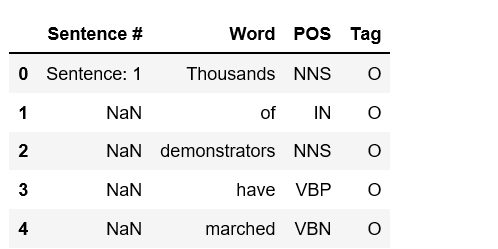Figure 2
`df.isnull().sum()`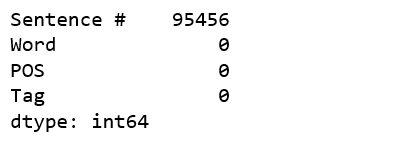Figure 3

### Data Preprocessing

We notice that there are many NaN values in ‘Sentence #” column, and we fill NaN by preceding values.

```df = df.fillna(method='ffill')

df['Sentence #'].nunique(), df.Word.nunique(), df.Tag.nunique()```

(4544, 10922, 17)

We have 4,544 sentences that contain 10,922 unique words and tagged by 17 tags.
The tags are not evenly distributed.

`df.groupby('Tag').size().reset_index(name='counts')`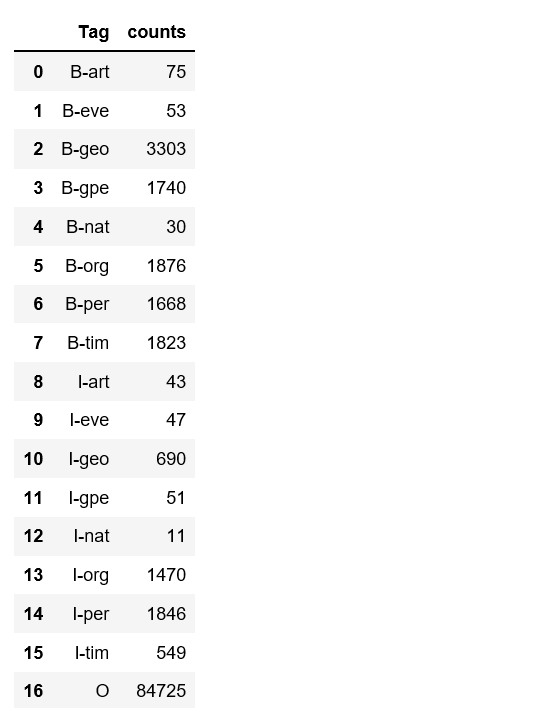Figure 4

The following code transform the text date to vector using `DictVectorizer`and then split to train and test sets.

```X = df.drop('Tag', axis=1)
v = DictVectorizer(sparse=False)
X = v.fit_transform(X.to_dict('records'))
y = df.Tag.values

classes = np.unique(y)
classes = classes.tolist()

X_train, X_test, y_train, y_test = train_test_split(X, y, test_size = 0.33, random_state=0)
X_train.shape, y_train.shape```

((67000, 15507), (67000,))

### Out-of-core Algorithms

We will try some of the out-of-core algorithms that are designed to process data that is too large to fit into a single computer memory that support `partial_fit` method.

Perceptron

```per = Perceptron(verbose=10, n_jobs=-1, max_iter=5)
per.partial_fit(X_train, y_train, classes)```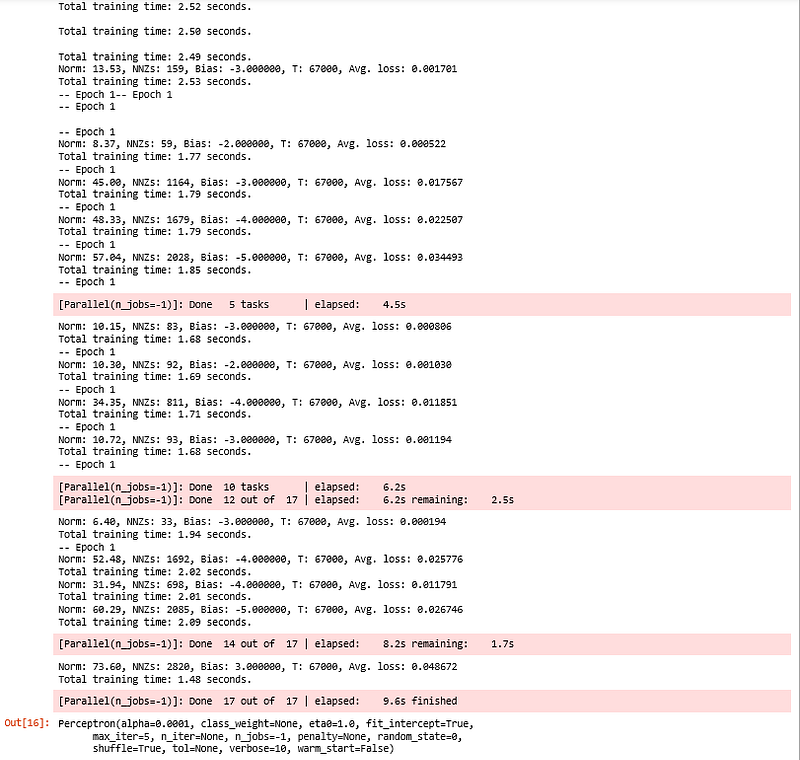Figure 5

Because tag “O” (outside) is the most common tag and it will make our results look much better than they actual are. So we remove tag “O” when we evaluate classification metrics.

```new_classes = classes.copy()
new_classes.pop()
new_classes```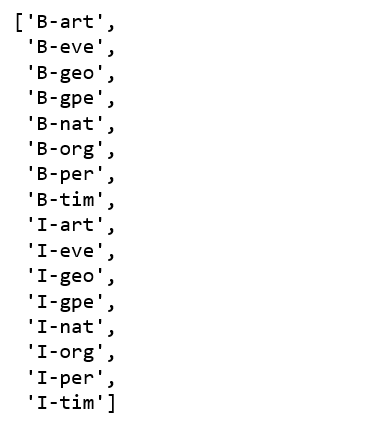Figure 6
`print(classification_report(y_pred=per.predict(X_test), y_true=y_test, labels=new_classes))`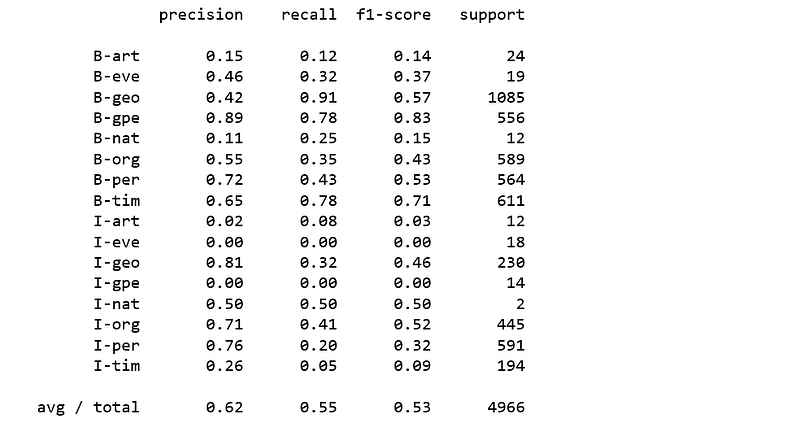Figure 7

Linear classifiers with SGD training

```sgd = SGDClassifier()
sgd.partial_fit(X_train, y_train, classes)```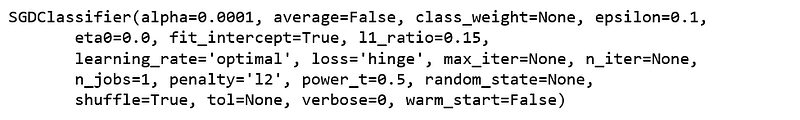Figure 8
`print(classification_report(y_pred=sgd.predict(X_test), y_true=y_test, labels=new_classes))`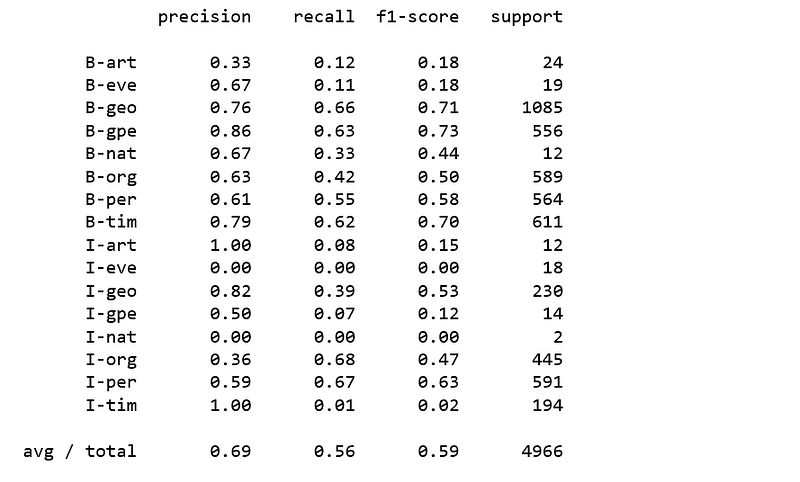Figure 9

Naive Bayes classifier for multinomial models

```nb = MultinomialNB(alpha=0.01)
nb.partial_fit(X_train, y_train, classes)```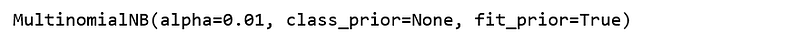Figure 10
`print(classification_report(y_pred=nb.predict(X_test), y_true=y_test, labels = new_classes))`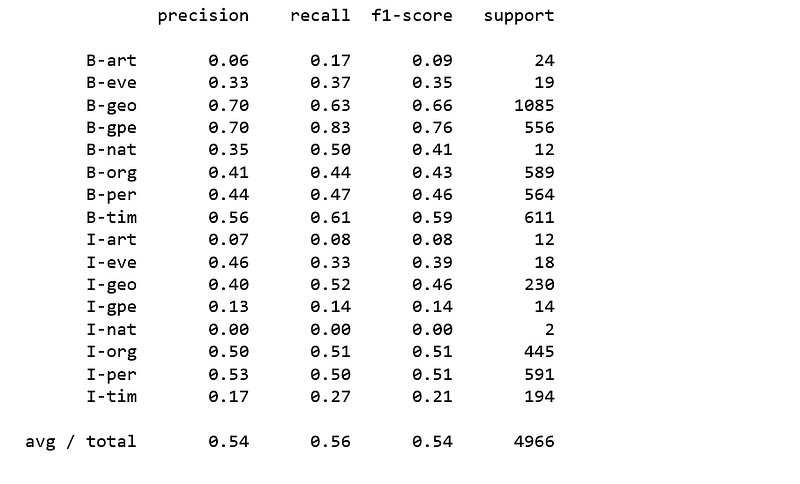Figure 11

Passive Aggressive Classifier

```pa =PassiveAggressiveClassifier()
pa.partial_fit(X_train, y_train, classes)```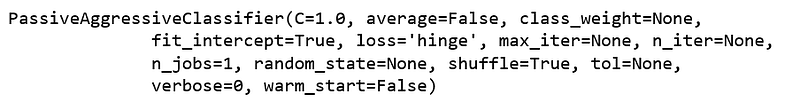Figure 12
`print(classification_report(y_pred=pa.predict(X_test), y_true=y_test, labels=new_classes))`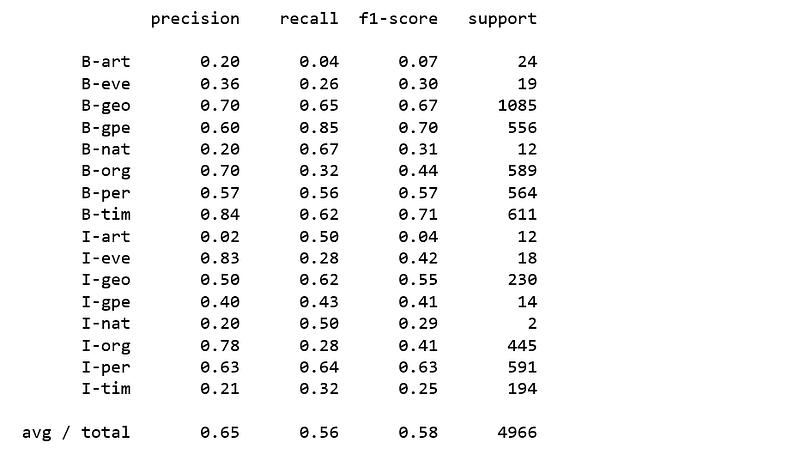Figure 13

None of the above classifiers produced satisfying results. It is obvious that it is not going to be easy to classify named entities using regular classifiers.